## 2.2 Spectral synthesis

Spectral synthesis (also called Fourier transform filtering) is another representative of how fBm surface can be obtained .

First step we compute the complex coefficientsof inverse Fourier transformation. In order to generate fBm spectral density function S(f) (c.f. ) of these coefficients must be proportional to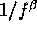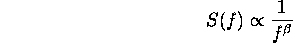wherecontrols the fractal dimension of the final object according to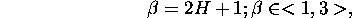and H denotes Hurst exponent. Then we calculate the inverse Fourier transformation in two dimensions according to formula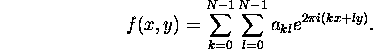The function f(x,y) is then fBm with its fractal dimensionThe coefficients of Fourier transformation with lowest indices have the biggest influence to resulting shape of the surface (see in Figure 4) and the higher indices manifest themselves just locally.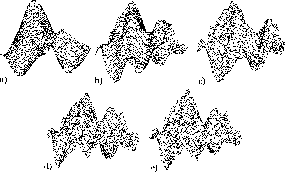Figure: Surfaces obtained with the spectral synthesis using a) 2, b) 4, c) 8, d) 16 and e) 32 respectively members of Fourier series.

Ivo Marak - marak@sgi.felk.cvut.cz